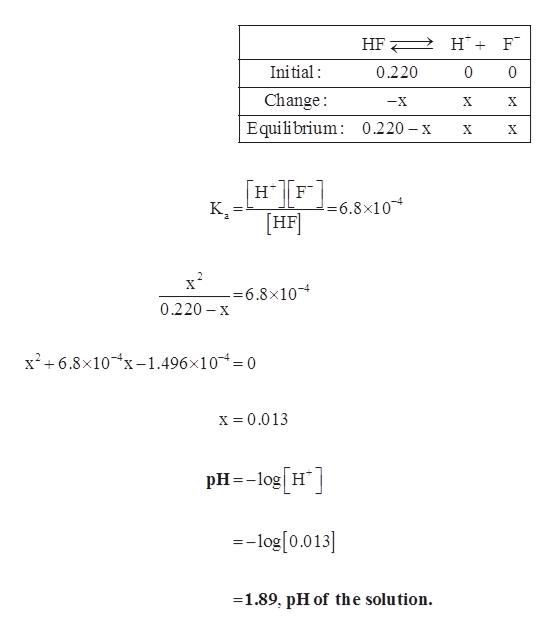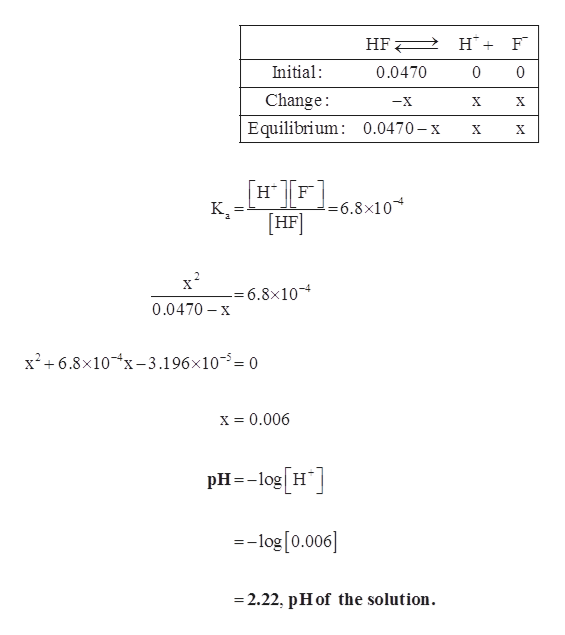# Determine the pH of an HF solution of each of the following concentrations. In which cases can you not make the simplifying assumption that x is small? (Ka for HF is 6.8×10−4.) a) 0.220 MExpress your answer to two decimal places.b) 0.0470 MExpress your answer to two decimal places.c) 0.0270 MExpress your answer to two decimal places.

Question

Determine the pH of an HF solution of each of the following concentrations. In which cases can you not make the simplifying assumption that x is small? (Ka for HF is 6.8×10−4.)

a) 0.220 M

b) 0.0470 M
c) 0.0270 M
check_circle

Step 1

(a)

ICE table for the reaction is given below.help_outlineImage TranscriptioncloseHF H F Initial 0.220 0 0 Change Equilibrium 0.220 -x -X X X X X н|F [HF !=6.8x10 к,- -=6.8x10 0.220 x x2+6.8x10-1.496x10= 0 x= 0.013 pHlogH --log[0.013 1.89, pH of the solution. fullscreen
Step 2

(b)

ICE table for the reactio...help_outlineImage TranscriptioncloseHF H F Initial: 0.0470 0 0 Change -X X X Equilibrium 0.0470-x X HF к- =6.8x10 HF -= 6.8x10 0.0470 x x26.8x10x-3.196x105= 0 x = 0.006 pHlog H --log [0.006] -2.22, pHof the solution. fullscreen

### Want to see the full answer?

See Solution

#### Want to see this answer and more?

Solutions are written by subject experts who are available 24/7. Questions are typically answered within 1 hour.*

See Solution
*Response times may vary by subject and question.
Tagged in

### Chemistry